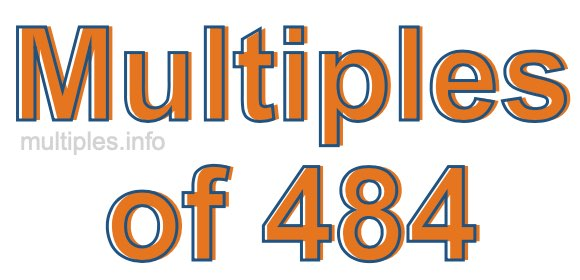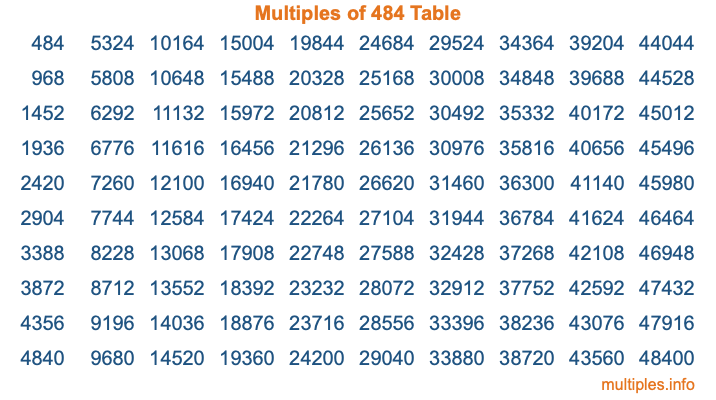Multiples of 484Welcome to the Multiples of 484 page. Here we will first teach you everything you will ever need to know about the multiples of 484, and then give you a study guide summary of everything we taught you to make sure you remember it all. Use this page to look up facts and learn information about the multiples of 484. This page will make you a multiples of four hundred eighty-four expert!

Definition of Multiples of 484
Multiples of 484 are all the numbers that when divided by 484 equal an integer. Each of the multiples of 484 are called a multiple. A multiple of 484 is created by multiplying 484 by an integer.

Therefore, to create a list of multiples of 484, you start with 1 multiplied by 484, then 2 multiplied by 484, then 3 multiplied by 484, and so on for as long as you want. Thus, the list of the first five multiples of 484 is 484, 968, 1452, 1936, and 2420. To see a larger list of multiples of 484, see the printable image of Multiples of 484 further down on this page. We also have a category where you can choose any nth multiple of 484.

Multiples of 484 Checker
The Multiples of 484 Checker below checks to see if any number of your choice is a multiple of 484. In other words, it checks to see if there is any number (integer) that when multiplied by 484 will equal your number. To do that, we divide your number by 484. If the the quotient is an integer, then your number is a multiple of 484.

Is  a multiple of 484?

Least Common Multiple of 484 and ...
A Least Common Multiple (LCM) is the lowest multiple that two or more numbers have in common. This is also called the smallest common multiple or lowest common multiple and is useful to know when you are adding our subtracting fractions. Enter one or more numbers below (484 is already entered) to find the LCM.

Check out our LCM Calculator if you need more details about the Least Common Multiple or if you need the LCM for different numbers for adding and subtraction fractions.

nth Multiple of 484
As we stated above, 484 is the first multiple of 484, 968 is the second multiple of 484, 1452 is the third multiple of 484, and so on. Enter a number below to find the nth multiple of 484.

th multiple of 484

Multiples of 484 vs Factors of 484
484 is a multiple of 484 and a factor of 484, but that is where the similarities end. All postive multiples of 484 are 484 or greater than 484. All positive factors of 484 are 484 or less than 484.

Below is the beginning list of multiples of 484 and the factors of 484 so you can compare:

Multiples of 484: 484, 968, 1452, 1936, 2420, etc.

Factors of 484: 1, 2, 4, 11, 22, 44, 121, 242, 484

As you can see, the multiples of 484 are all the numbers that you can divide by 484 to get a whole number. The factors of 484, on the other hand, are all the whole numbers that you can multiply by another whole number to get 484.

It's also interesting to note that if a number (x) is a factor of 484, then 484 will also be a multiple of that number (x).

Multiples of 484 vs Divisors of 484
The divisors of 484 are all the integers that 484 can be divided by evenly. Below is a list of the divisors of 484.

Divisors of 484: 1, 2, 4, 11, 22, 44, 121, 242, 484

The interesting thing to note here is that if you take any multiple of 484 and divide it by a divisor of 484, you will see that the quotient is an integer.

Multiples of 484 Table
Below is an image of the first 100 multiples of 484 in a table. The table is in chronological order, column by column. The first column has the first ten multiples of 484, the second column has the next ten multiples of 484, and so on.The Multiples of 484 Table is also referred to as the 484 Times Table or Times Table of 484. You are welcome to print out our table for your studies.

Negative Multiples of 484
Although not often discussed or needed in math, it is worth mentioning that you can make a list of negative multiples of 484 by multiplying 484 by -1, then by -2, then by -3, and so on, to get the following list of negative multiples of 484:

-484, -968, -1452, -1936, -2420, etc.

Multiples of 484 Summary
Below is a summary of important Multiples of 484 facts that we have discussed on this page. To retain the knowledge on this page, we recommend that you read through the summary and explain to yourself or a study partner why they hold true.

There are an infinite number of multiples of 484.

A multiple of 484 divided by 484 will equal a whole number.

484 divided by a factor of 484 equals a divisor of 484.

The nth multiple of 484 is n times 484.

The largest factor of 484 is equal to the first positive multiple of 484.

484 is a multiple of every factor of 484.

484 is a multiple of 484.

A multiple of 484 divided by a divisor of 484 equals an integer.

484 divided by a divisor of 484 equals a factor of 484.

Any integer times 484 will equal a multiple of 484.

Multiples of a Number
Here you can get the multiples of another number, all with the same attention to detail as we did for multiples of 484 on this page.

Multiples of
Multiples of 485
Did you find our page about multiples of four hundred eighty-four educational? Do you want more knowledge? Check out the multiples of the next number on our list!